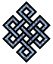fail2ban bad ip database: ip 122.175.55.196

| ip database | live view | stats | report | help | api key:

 ip: 122.175.55.196 hostname: abts-ap-static-196.55.175.122.airtelbroadband.in country:[IN] India first reported: 21.11.2018 05:04.45 GMT+0200 last reported: 05.03.2019 04:11.46 GMT+0200 time period: 103d 23h 07m 01s total reports: 12 reported by: 2 host(s) filter(s): ssh (7) ssh (5) tor exit node no badips.com db Lookupport scan of '122.175.55.196':

[-hide]
```# Nmap 6.40 scan initiated Wed Nov 21 05:05:03 2018 as: /usr/bin/nmap -sU -sS -O 122.175.55.196
Nmap scan report for abts-ap-static-196.55.175.122.airtelbroadband.in (122.175.55.196)
Host is up (0.14s latency).
Not shown: 1000 open|filtered ports, 989 filtered ports
PORT     STATE  SERVICE
22/tcp   open   ssh
80/tcp   open   http
443/tcp  closed https
1521/tcp open   oracle
3389/tcp closed ms-wbt-server
3390/tcp open   dsc
5901/tcp closed vnc-1
5902/tcp closed vnc-2
8009/tcp closed ajp13
8080/tcp open   http-proxy
9999/tcp closed abyss
No exact OS matches for host (If you know what OS is running on it, see http://nmap.org/submit/ ).
TCP/IP fingerprint:
OS:SCAN(V=6.40%E=4%D=11/21%OT=22%CT=443%CU=%PV=N%G=Y%TM=5BF4DA25%P=x86_64-p
OS:c-linux-gnu)SEQ(SP=104%GCD=1%ISR=10E%TI=Z%TS=A)SEQ(SP=104%GCD=1%ISR=10E%
OS:TI=Z%CI=RD%TS=A)OPS(O1=M5B4ST11NW7%O2=M5B4ST11NW7%O3=M5B4NNT11NW7%O4=M5B
OS:4ST11NW7%O5=M5B4ST11NW7%O6=M5B4ST11)WIN(W1=7120%W2=7120%W3=7120%W4=7120%
OS:W5=7120%W6=7120)ECN(R=Y%DF=Y%TG=40%W=7210%O=M5B4NNSNW7%CC=Y%Q=)T1(R=Y%DF
OS:=Y%TG=40%S=O%A=S+%F=AS%RD=0%Q=)T2(R=N)T3(R=N)T4(R=Y%DF=Y%TG=40%W=0%S=A%A
OS:=Z%F=R%O=%RD=0%Q=)T5(R=Y%DF=Y%TG=40%W=0%S=Z%A=S+%F=AR%O=%RD=0%Q=)T6(R=Y%
OS:DF=Y%TG=40%W=0%S=A%A=Z%F=R%O=%RD=0%Q=)T7(R=Y%DF=Y%TG=40%W=0%S=Z%A=S+%F=A
OS:R%O=%RD=0%Q=)U1(R=N)IE(R=Y%DFI=S%TG=20%CD=S)

OS detection performed. Please report any incorrect results at http://nmap.org/submit/ .
# Nmap done at Wed Nov 21 05:08:05 2018 -- 1 IP address (1 host up) scanned in 183.36 seconds
```
```Σ = 66 | Δt = 0.0040199756622314s
```